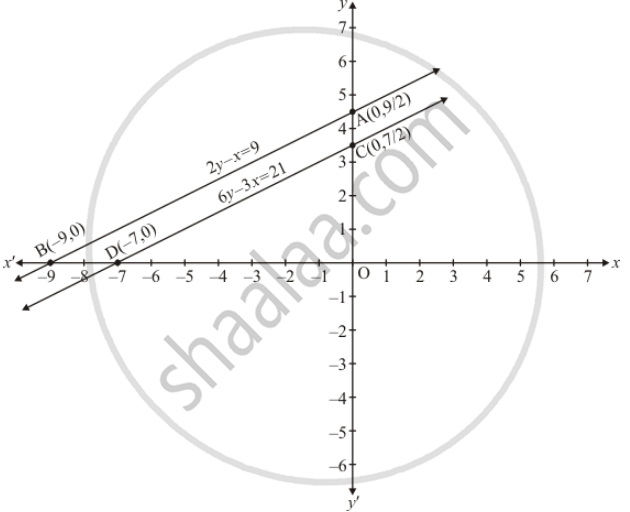Share

# Show Graphically that Each One of the Following Systems of Equations is Inconsistent (I.E. Has No Solution) : 2y − X = 9 6y − 3x = 21 - CBSE Class 10 - Mathematics

ConceptGraphical Method of Solution of a Pair of Linear Equations

#### Question

Show graphically that each one of the following systems of equations is inconsistent (i.e. has no solution) :

2y − x = 9

6y − 3x = 21

#### Solution

The given equations are

2y - x = 9 ...(i)

6y  - 3x = 21 ....(ii)

Putting x = 0 in equation (i) we get

=> 2y - 0 = 9

=> y = 9/2

x = 0, y = 9/2

Putting y= 0 in equation (i) weget

=> 2 xx -x = 9

=> x = -9

=> x = -9, y = 0

Use the following table to draw the graph.

 x 0 -9 y 9/2 0

Draw the graph by plotting the two points A(0, 9/2), B(-9,0) from table6y - 3x = 21 ...(ii)

Putting x = 0 in equation (ii) we get

=> 6y - 3 xx 0 = 21

=> y = 7/2

=> x = 0, y = 7/2

Putting y = 0 in equation (ii) we get

=> 6 xx 0 - 3x = 21

=> x = -7

:. x = -7, y= 0

Use the following table to draw the graph.

 x 0 -7 y 7/2 0

Draw the graph by plotting the two points C(0, 7/2), D(-7,0) from table.

Here two lines are parallel and so don’t have common points

Hence the given system of equations has no solution.

Is there an error in this question or solution?

#### APPEARS IN

Solution Show Graphically that Each One of the Following Systems of Equations is Inconsistent (I.E. Has No Solution) : 2y − X = 9 6y − 3x = 21 Concept: Graphical Method of Solution of a Pair of Linear Equations.
S